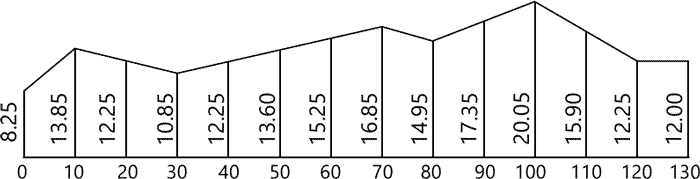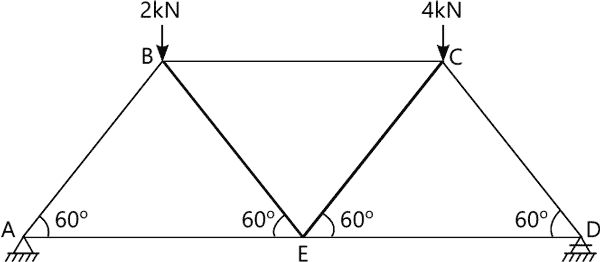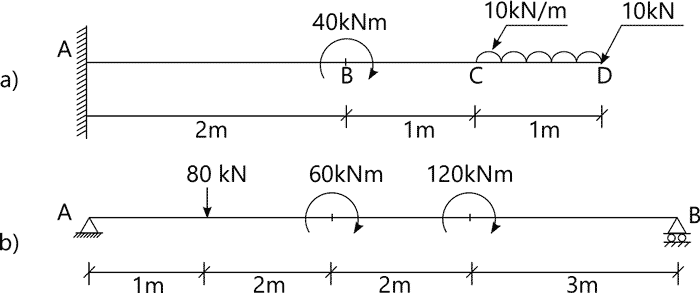MORE IN Basic Civil Engg. & Engg. Mechanics
RGPV First Year Engineering (Set B) (Semester 1)
Basic Civil Engg. & Engg. Mechanics
December 2013
Total marks: --
Total time: --
INSTRUCTIONS
(1) Assume appropriate data and state your reasons
(2) Marks are given to the right of every question
(3) Draw neat diagrams wherever necessary

Answer any one question from Q1 & Q2
1 (a) What is concrete and how is it made? Also mention factors affecting strength of concrete.
7 M
1 (b) Describe briefly the tests to which bricks may be put before using them for engineering purposes.
7 M

2 (a) What are the requirements of a good stair? Discuss them in detail.
7 M
2 (b) Give a list of materials which are commonly used as floorings and give a brief description of each material.
7 M

Answer any one question from Q3 & Q4
3 The following consecutive staff readings were taken with a level and 3m leveling staff on a continuously slopping ground at a common interval of 20m:0.605, 1.235, 1.860, 2.575, 0.240,0.915, 1.935, 2.875, 1.825, 2.725. The R.L of the first point was 192.120. Rule a page of a level field book and enter the above readings. Calculate the reduced levels of the points and also the gradient of the line joining the first and last points.
14 M

4 (a) What is the basic difference between temporary and permanent adjustments of a theodolite?
7 M
4 (b) What are the different methods of plane tabling? Describe the one of them fully with neat sketches.
7 M

Answer any one question from Q5 & Q6
5 (a) With reference to civil engineering practice explain what is meant by the following:
i) Trapezoidal and Prismoidal rules.
ii) Prismoidal corrections.
7 M
5 (b) Describe briefly the remote sensing and its applications.
7 M

6 The following perpendicular offsets in mare measured from a straight line to an irregular boundaryat regular intervals of 10m.
h1=8.25, h2=13.85, h3=12.25, h4=10.85, h5=12.25, h6=13.60, h7=15.25, h8=16.85, h9=14.95, h10=17.35, h11=20.05, h12=15.90, h13=12.25, h14=12.00. Compute the area included between the straight line, the irregular boundary byi) Trapezoidal rule,
ii) Simpson?s one-third rule (a) using h1as the first offset, (b) using h14as the first offset.
14 M

Answer any one question from Q7 & Q8
7 (a) Obtain an expression for minimum force required to move a body on a horizontal plane.
7 M
7 (b) A block of 300N weight is resting on a plane inclined at 30° to the horizontal, the coefficient of friction between the block and the plane is 0.4. A force, inclined at an angle of 30°is acting on the block. Find the minimum force to move the block upwards.
7 M

8 The length of each member of the truss shown in Fig.1 is 4m. Loads of 2kN and 4kN are applied at joints B and C of the truss. Determine the magnitudes and nature of forces in all members of the truss. Both ends are simply supported.14 M

Answer any one question from Q9 & Q10
9 For a circular pipe of 150 mm external diameter and 120 mm internal diameter, determine.
(i) moment of inertia about its diameter
(ii) the radius of gyration and
(iii) the polar moment of inertia.
14 M

10 Draw the shear force and bending moment diagrams for the beams shown in Fig.2
:14 M

More question papers from Basic Civil Engg. & Engg. Mechanics